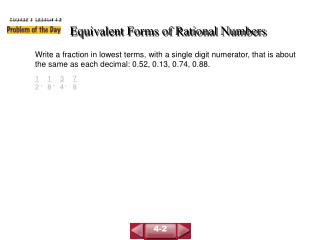DownloadDownload PresentationEquivalent Forms of Rational Numbers

# Equivalent Forms of Rational Numbers

Download Presentation## Equivalent Forms of Rational Numbers

- - - - - - - - - - - - - - - - - - - - - - - - - - - E N D - - - - - - - - - - - - - - - - - - - - - - - - - - -
##### Presentation Transcript

1. 1 2 1 8 3 4 7 8 , , , COURSE 3 LESSON 4-2 Equivalent Forms of Rational Numbers Write a fraction in lowest terms, with a single digit numerator, that is about the same as each decimal: 0.52, 0.13, 0.74, 0.88. 4-2

2. 1 4 0.25 The remainder is 0. quotient 1 4 0.25 or 1 ÷ 4 = 41.00 –8 20 –20 0 1 4 1 4 So, = 0.25. The total amount of rainfall was 0.25 in. COURSE 2 LESSON 3-9 Fractions and Decimals The total amount of rainfall yesterday was reported as in. Express the amount of rainfall as a decimal. Method 2  Calculator Method 1  Paper and Pencil 3-9

3. 7 15 7 15 7 15 0.4666 7 15 0.46666667 There will always be a remainder. The digit “6” repeats. or 7 ÷ 15 = 157.0000 –60 100 –90 100 –90 10 So, = 0.46. COURSE 2 LESSON 3-9 Fractions and Decimals Write as a decimal. Method 1  Paper and Pencil Method 2  Calculator 3-9

4. 3,225 1,000 3.225 1 3.225 = Write as a fraction with the denominator 1. Since there are 3 digits to the right of the decimal, multiply the numerator and denominator by 103 or 1,000. = 3,225 ÷ 25 1,000 ÷ 25 = = Simplify using the GCF, 25. 9 40 129 40 Write as a mixed number. = 3 COURSE 3 LESSON 4-2 Equivalent Forms of Rational Numbers Write 3.225 as a mixed number. 4-2

5. Since 0.105 = , 4.105 = 4 . 105 1,000 105 1,000 105 1,000 105 ÷ 5 1,000 ÷ 5 Use the GCF to write the fraction in simplest form. 4 = 4 = 4 21 200 COURSE 2 LESSON 3-9 Fractions and Decimals Write 4.105 as a fraction in simplest form. 3-9

6. walk = 0.2083 Use a calculator to change the fractions to decimals. bicycles = 0.0625 5 24 1 16 1 16 5 24 Since 0.375 > 0.25 > 0.2083 > 0.0625, the means of transportation are car, bus, walking, and bicycle. COURSE 2 LESSON 3-9 Fractions and Decimals In a survey of next year’s seventh-grade students, 0.25 said they will come to school by bus, said they will walk, 0.375 said they will come in a car, and said they will ride their bicycles. Order the means of transportation from most used to least used. 3-9

7. Step 1 Let the variable n represent the given decimal. n = 0.23 Step 2 Since 2 digits repeat, multiply each side by 102, or 100. Step 3 Subtract to eliminate the repeating part, 0.23. 100n = 23.23 100n = 23.232323 . . . Use the Subtraction Property of Equality. – n = – 0.232323 . . . 99n = 23.000000 Simplify. 99n = 23 COURSE 3 LESSON 4-2 Equivalent Forms of Rational Numbers Write the repeating decimal 0.23 as a fraction in simplest form. 4-2

8. = Divide each side by 99. 23 99 23 99 23 99 99n 99 n = Simplify. The repeating decimal 0.23 equals . Check Use a calculator to divide 23 by 99. 23 99 0.23232323 COURSE 3 LESSON 4-2 Equivalent Forms of Rational Numbers (continued) Step 4 Solve the new equation. 4-2

9. 7 8 4 9 5 16 3 5 0.4 COURSE 2 LESSON 3-9 Fractions and Decimals Write each fraction as a decimal. 1. 2. 3.4. 0.875 0.6 0.3125 3-9

10. 2 3 3 4 – 2 25 99 7 COURSE 3 LESSON 4-2 Equivalent Forms of Rational Numbers Write each as a fraction in simplest form. 1.2. – 3. 2.75 4. 0.2 5. 0.4 6. 7.25 12 18 30 42 5 7 2 9 2 5 4-2